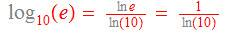# Taylor series for log(x)

• I
• PainterGuy

#### PainterGuy

Hi,

I was trying to solve the following problem myself but couldn't figure out how the given Taylor series for log(x) is found.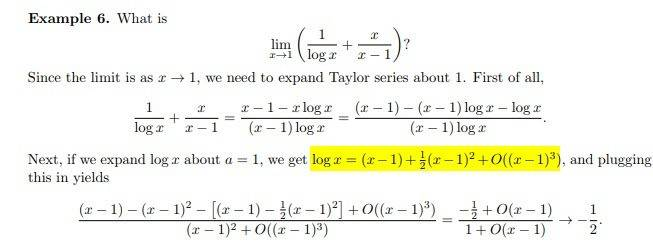Taylor series for a function f(x) is given as follows.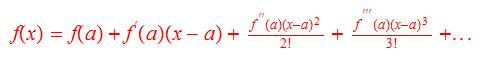Question 1:
I was trying to find the derivative of log(x).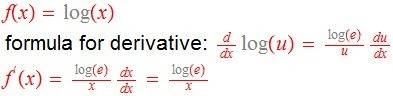My calculator gives it as d{log(x)}/dx=1/In(x)*x.

How do I convert log(e)/x to 1/In(x)*x? Logarithm base change formula isn't working for me. Thank you for your help!

#### Attachments

Last edited:

The log function in the example is the natural log, so log(e) = 1.

•PainterGuy
Thank you!

Question 1:
The log function in the example is the natural log, so log(e) = 1.

I believe that you are referring to Example 6 shown below.You can see that the Taylor series in Example 6 doesn't have "x" and "x²" in denominator for the first two terms. But the Taylor series found by me has "x" and "x²" in denominator as shown below. Where am I going wrong?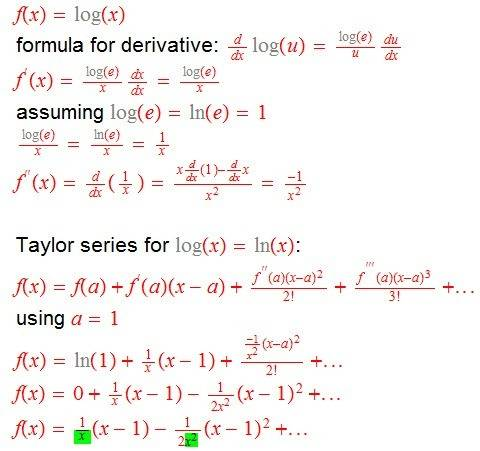Question 2: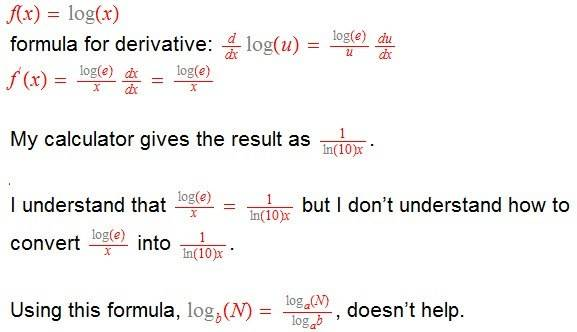#### Attachments

Thank you!

Question 1:

I believe that you are referring to Example 6 shown below.

View attachment 245372

You can see that the Taylor series in Example 6 doesn't have "x" and "x²" in denominator for the first two terms. But the Taylor series found by me has "x" and "x²" in denominator as shown below. Where am I going wrong?

View attachment 245373

I think you've calculated f'(x) = 1/x and f''(x) = -1/x^2, but forgot that these derivatives are evaluated at x = a, and should therefore be 1/a = 1 and -1/a^2 = -1.

•PainterGuy
On your calculator, "log" means ##\log_{10}## whereas in the example "log" means ##\log_e## or what on your calculator is called ##\ln##. It's pretty common in mathematics to dispense with the ##\ln## notation and use ##\log## to refer to the natural logarithm.

•PainterGuy
Thanks a lot!

I understand it now.

The following is a related example with a=2.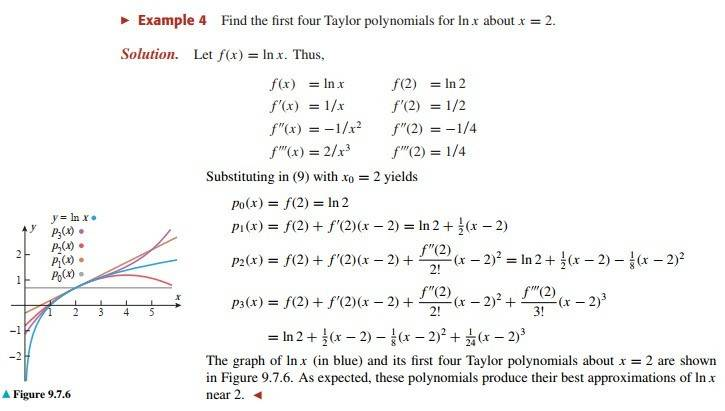Also: